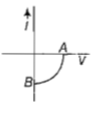The given graph represents V-I characteristic for a semiconductor device. Which of the following statement is correct?(a) It is V-I characteristic for solar cell where point A represents open circuit voltage and point B short circuit current

(b) It is for a solar cell and points A and B represent open circuit voltage and current, respectively

(c) It is for a photodiode and points A and B represent open circuit voltage and current, respectively

(d) It is for a LED and points A and B represents open circuit voltage and short circuit current respectively

Explanation is a part of a Paid Course. To view Explanation Please buy the course.

Difficulty Level: